IT数码 购物 网址 头条 软件 日历 阅读 图书馆
 TxT小说阅读器 ↓语音阅读,小说下载,古典文学↓ 图片批量下载器 ↓批量下载图片,美女图库↓ 图片自动播放器 ↓图片自动播放器↓ 一键清除垃圾 ↓轻轻一点,清除系统垃圾↓
 开发: C++知识库 Java知识库 JavaScript Python PHP知识库 人工智能 区块链 大数据 移动开发 嵌入式 开发工具 数据结构与算法 开发测试 游戏开发 网络协议 系统运维 教程: HTML教程 CSS教程 JavaScript教程 Go语言教程 JQuery教程 VUE教程 VUE3教程 Bootstrap教程 SQL数据库教程 C语言教程 C++教程 Java教程 Python教程 Python3教程 C#教程 数码: 电脑 笔记本 显卡 显示器 固态硬盘 硬盘 耳机 手机 iphone vivo oppo 小米 华为 单反 装机 图拉丁
 -> 人工智能 -> 【GNN】第八章 图表征学习 -> 正文阅读

### [人工智能]【GNN】第八章 图表征学习

【GNN】第一章 图论基础

【GNN】第二章 PyG中的图与图数据集

【GNN】第三章 消息传递范式与PyG的MessagePassing基类

【GNN】第四章 节点表征学习与节点分类任务（理论+调包实操）

【GNN】第五章 构造数据完全存于内存的数据集类InMemoryDataset

【GNN】第六章 边预测任务

# 前言

• 图表征学习要求根据节点属性、边和边的属性（如果有的话）生成一个向量作为图的表征，基于图表征我们可以做图的预测
• 基于图同构网络（Graph Isomorphism Network, GIN）的图表征网络是当前最经典的图表征学习网络
• 通过对目前比较流行的 GNN 变体（如 GCN、GraphSAGE 等）进行分析，结果表明目前的 GNN 变体无法区分某些简单的图结构
• 这些 GNN 的设计主要是基于经验而谈，并没有很好的理论基础来分析 GNN 的性质和局限性。因此提出了分析 GNN 能力的理论框架
• 证明出 WL-test 是 GNN 的表达能力上限
• 设计了一个简单的架构 GIN，并证明该架构在目前所有 GNN 算法中最具表达能力，且具有与 Weisfeiler-Lehman 图同构测试一样强大的功能

# 1 WL-test

## 1.1 同构图 Graph Isomorphism

• 两图的边和顶点数量相同，且边的连接性相同
• 也可以认为一图的点是由另一图的点映射得到
• 计算图同构可以度量图的相似度（比如实际应用中具有相似结构的分子可能具备相似的功能特性）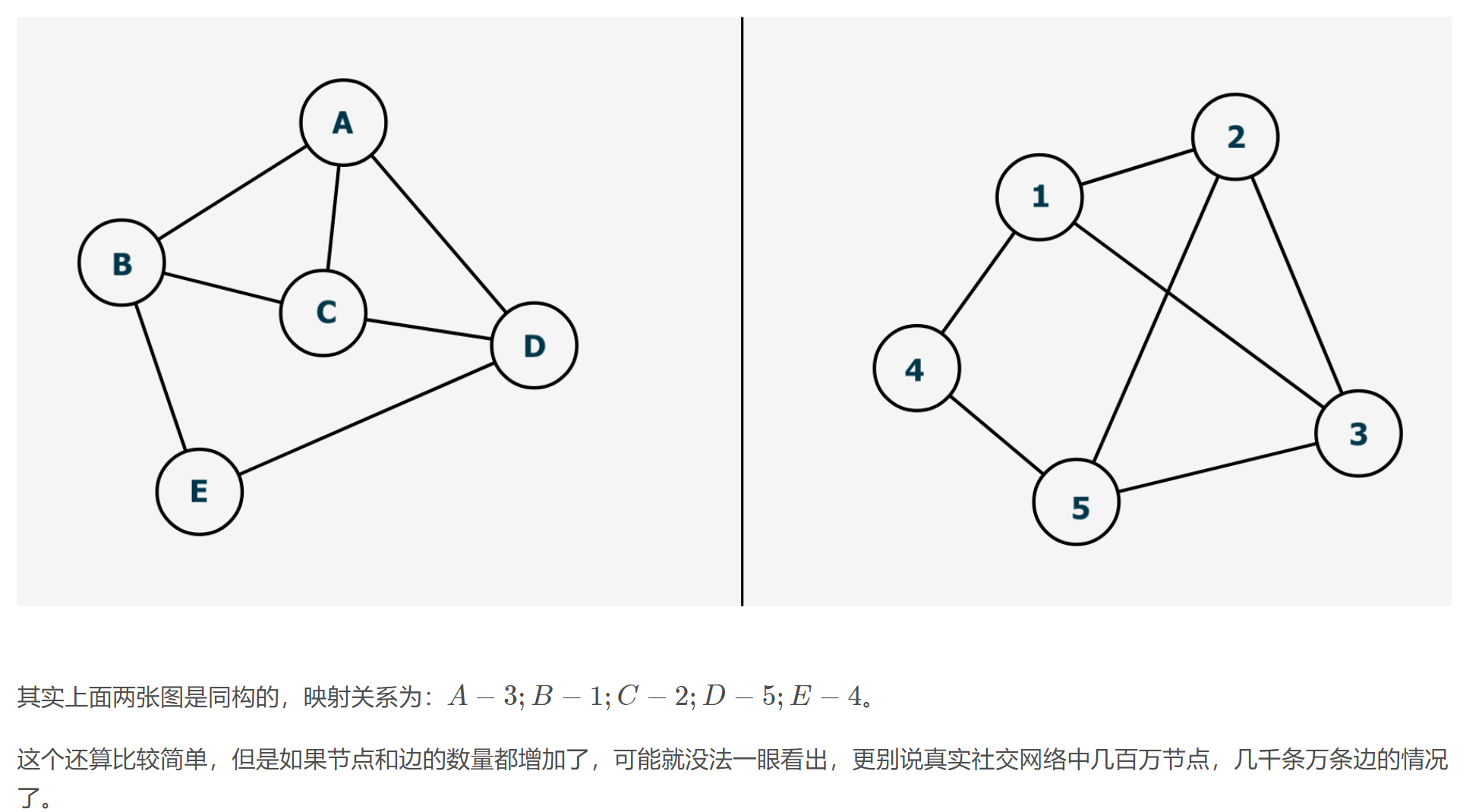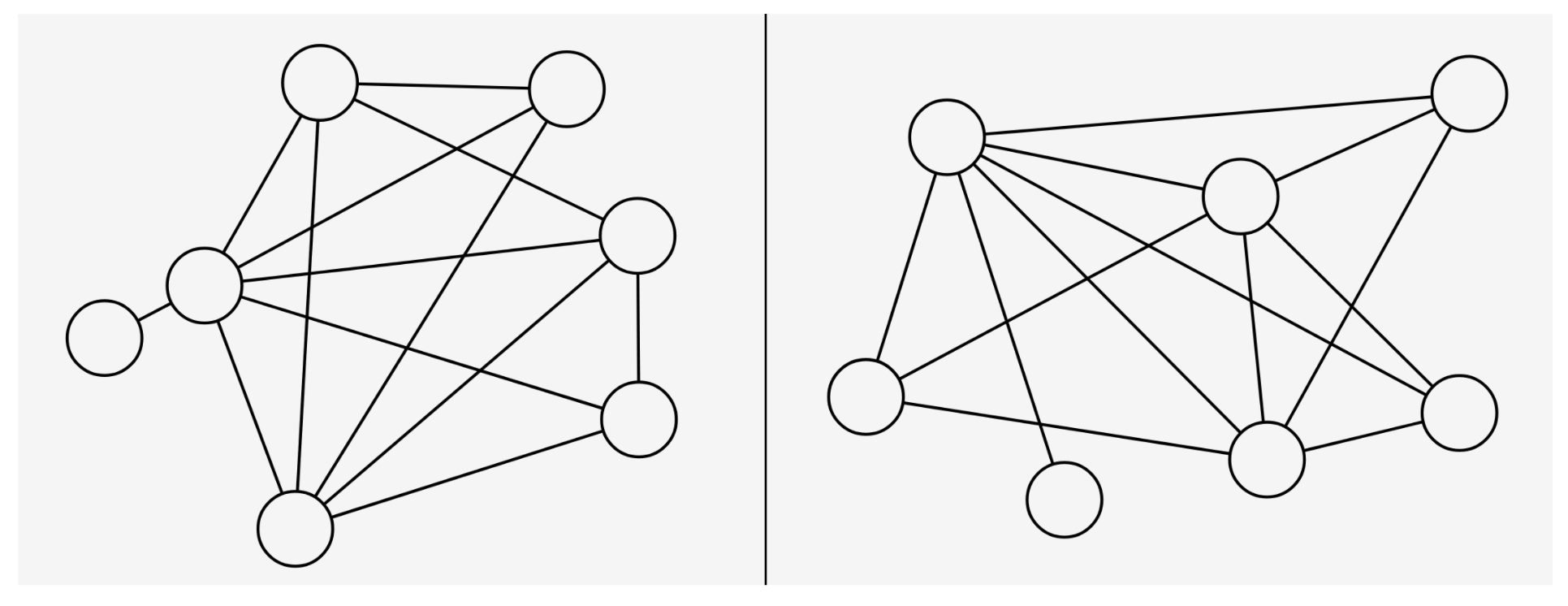## 1.3 1-dimensional WL-test 及图相似度量

• 通过计算图特征向量来衡量图相似度。
• WL 算法可以是 K-维的，K-维 WL 算法在计算图同构问题时会考虑顶点的 k 元组。如果只考虑顶点的自身特征（如标签、颜色等），那么就是 1-维 WL 算法
• 举例说明：一次迭代
1. 给定两个图 G G G ′ G^{\prime} ，每个节点拥有标签（实际中，一些图没有节点标签，我们可以以节点的度作为标签）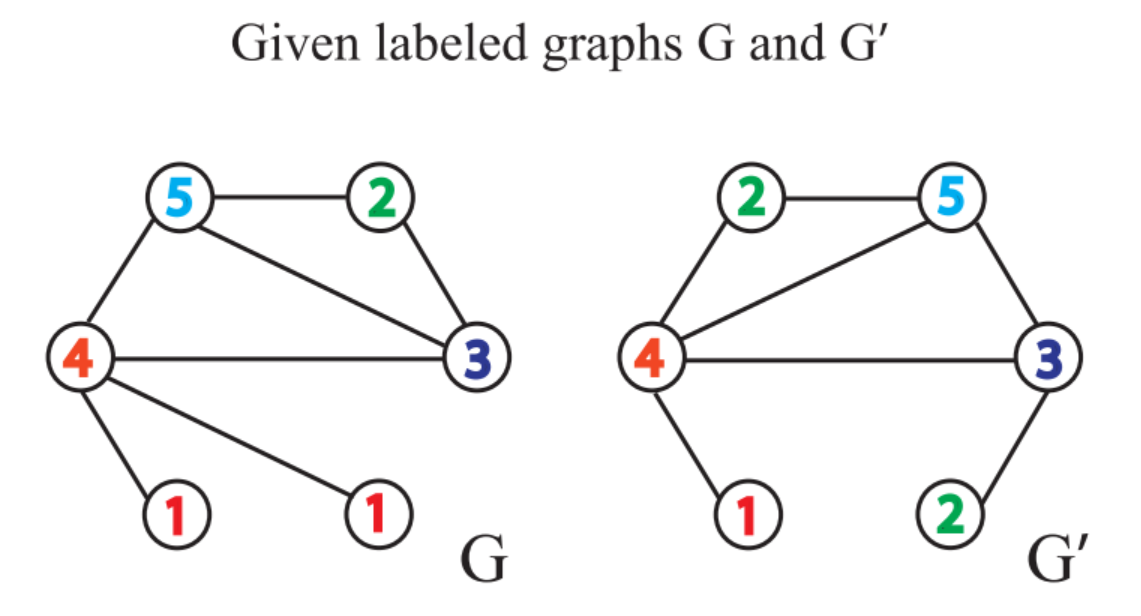2.3. 考虑节点邻域的标签，并对此排序。逗号前是当前标签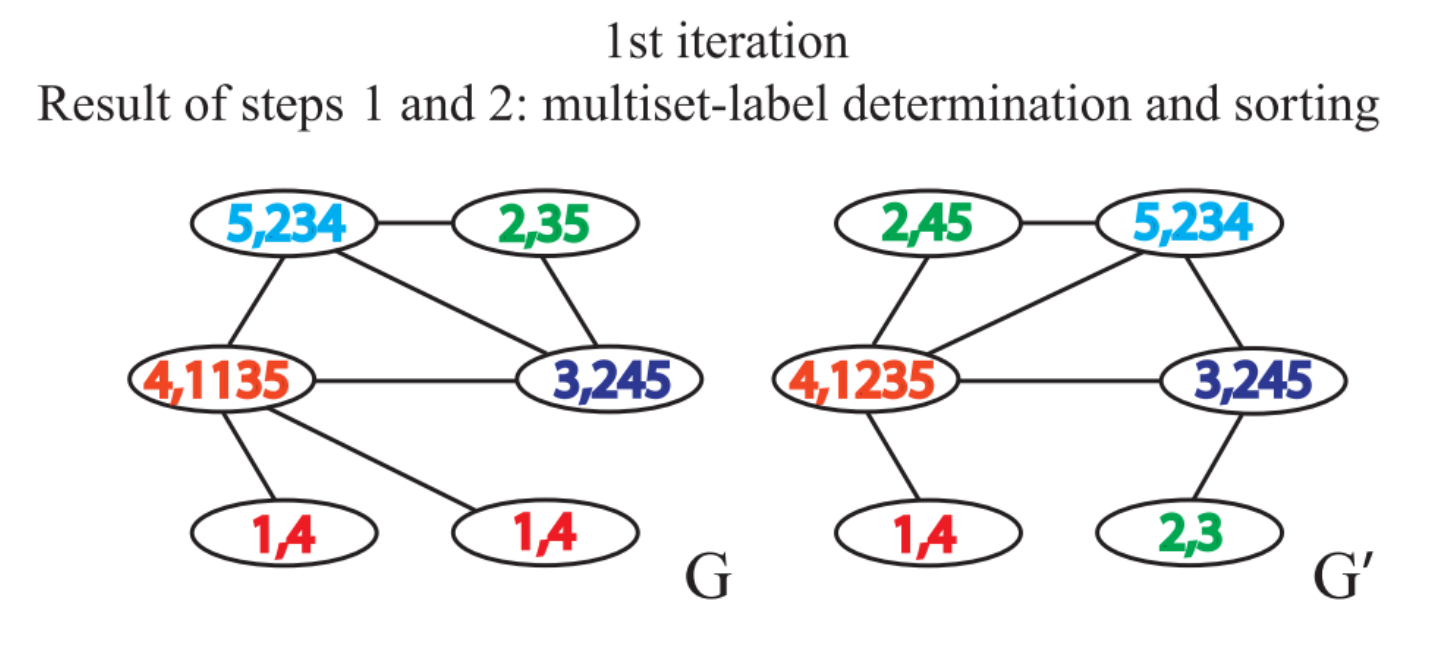4. 对标签进行压缩映射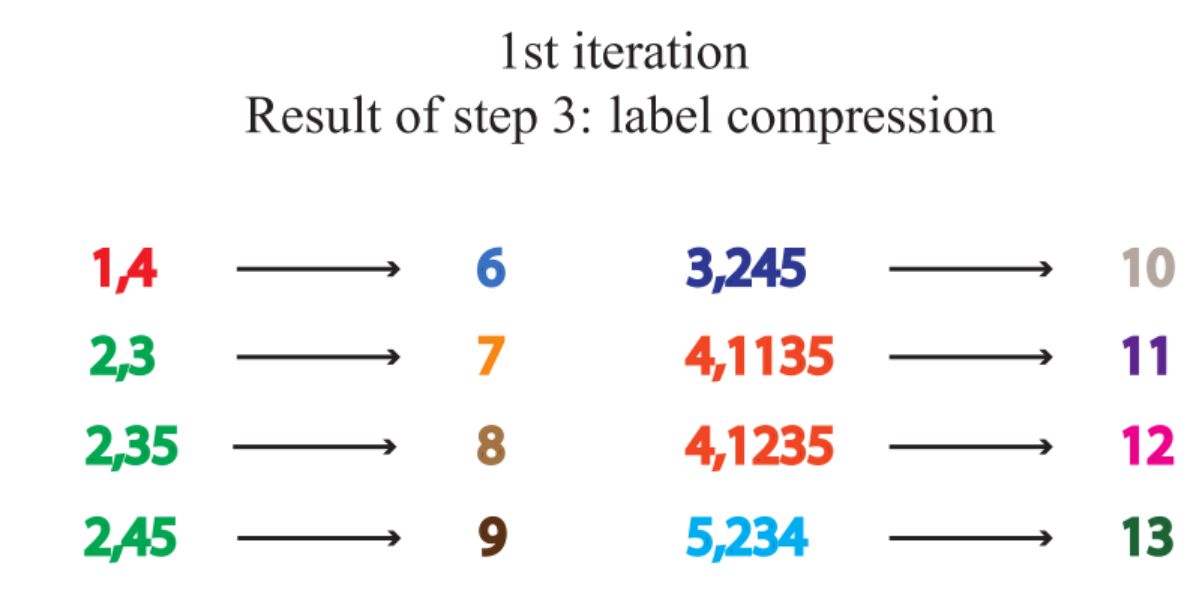5. 得到新的标签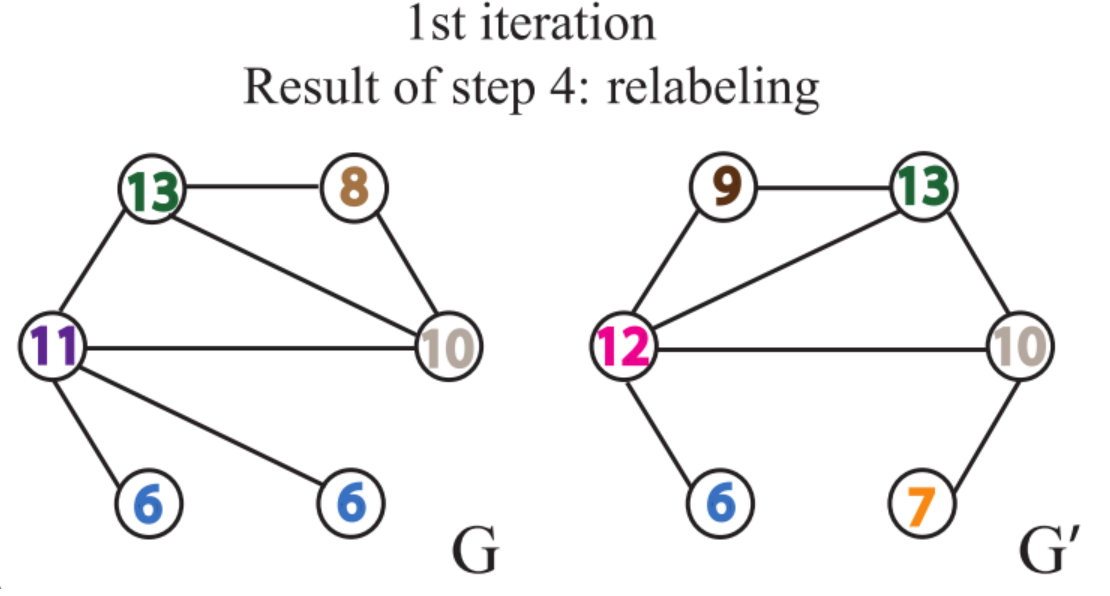6. 计算图特征向量：迭代 1 轮后，利用计数函数分别得到两张图的计数特征，得到图特征向量后便可计算图之间的相似性了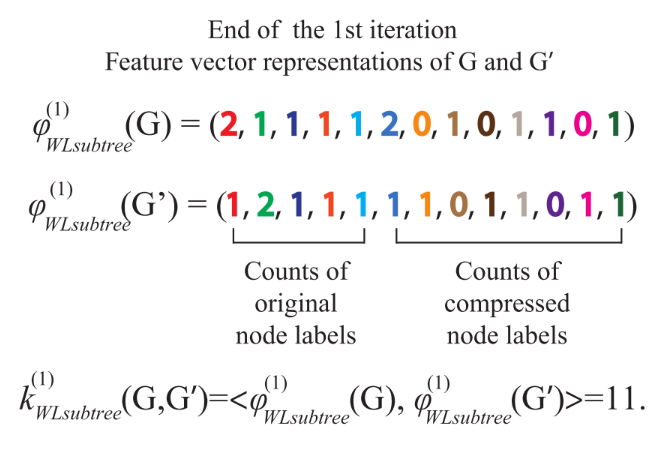• 当出现两个图相同节点标签的出现次数不一致时，即可判断两个图不相似
• 如果上述的步骤重复一定的次数后，没有发现有相同节点标签的出现次数不一致的情况，那么我们无法判断两个图是否同构

## 1.4 WL子树

• 在WL Test的第 k k 次迭代中，一个节点的标签代表了：以该节点为根的高度为 k k 的子树结构
• 当两个节点的 h h 层的标签一样时，表示：分别以这两个节点为根节点的WL子树是一致的
• 举例：右图是节点1迭代两次的子树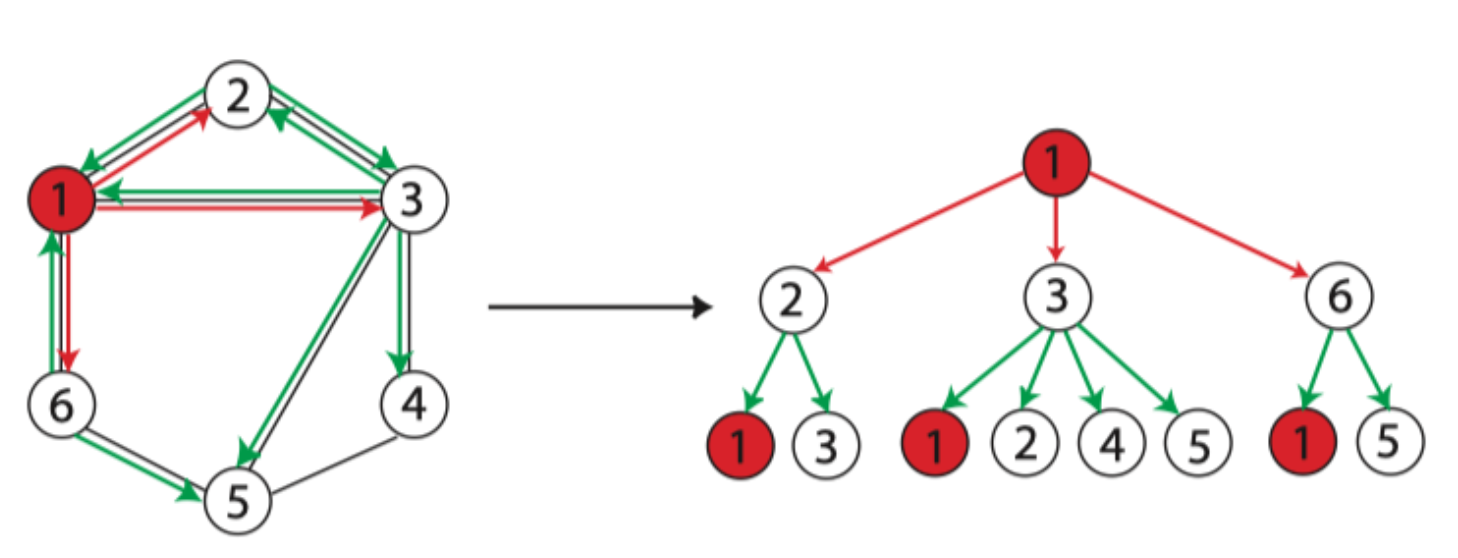## 1.5 WL-test的公式表示

• WL-test分为四步：聚合邻接节点标签、多重集排序、标签压缩、更新标签
• 公式：
a v k = f ( { h u k ? 1 : u ∈ N ( v ) } ) h v k = Hash ? ( h v k ? 1 , a v k ) a^{k}_v = f\left(\{ h^{k-1}_u:u\in N(v) \} \right) \\ h^{k}_{v} =\operatorname{Hash}\left(h_v^{k-1}, a_v^k\right)
• 由公式发现，WL-test和GNN一样，也分为两步：聚合和结合

# 2 GIN

## 2.1 单射的聚合方案

• 直观来说，一个好的 GNN 算法仅仅会在两个节点具有相同子树结构时才会将其映射到同一位置
• 由于子树结构是通过节点邻域递归定义的，所以我们可以将分析简化为这样一个问题：GNN 是否会映射两个邻域（即multiset）到相同的 Representation
• 一个好的 GNN 永远不会将两个不同领域映射得到相同的Representation。即，聚合模式必须是单射
• 因此，我们可以将 GNN 的聚合方案抽象为一类神经网络可以表示的多重集函数，并分析其是否是单射。

## 2.1 WL-test是GNN的上界

• 引理：
• 对于两个非同构图 G 1 G_1 G 2 G_2 ，如果存在一个图神经网络将这两个图映射到不同的Embedding向量中，那么也可以通过WL-test确定 G 1 G_1 G 2 G_2 是非同构图
• 反证法可以证明

## 2.2 GIN：一个与 WL-test 性能相当的 GNN

GNN 和 WL-test 的主要区别在于单射函数中。顺利成章的，作者设计一个满足单射函数的图同构网络
h v k = ? ( h v k ? 1 , f ( { h u k ? 1 : u ∈ N ( v ) } ) ) h_v^k = \phi(h^{k-1}_v,f(\{h_u^{k-1}:u\in N(v)\}))

• f f 作用在 multisets 上， ? \phi 为单射函数
• GNN 作用在 multiset 的 READOUT 函数也是单射的

### 2.2.1 聚合函数 Aggregate

• 引理：设 X \mathcal{X} （有限多重集的集合）可数，那么会存在一个函数 f f X → R n \mathcal{X}\rightarrow \mathbb{R}^n 使得对于任意有限多重集 X ? X X \subset \mathcal{X} 都有
h ( X ) = ∑ x ∈ X f ( x ) h(X) = \sum_{x\in X}f(x)
其中 h ( X ) h(X) 对各 X ? X X \subset \mathcal{X} 唯一
此外，任意一个多重集函数 g g 都可以被分解为：
g ( X ) = ? ( ∑ x ∈ X f ( x ) ) g(X) = \phi(\sum_{x\in X}f(x))
• 推论：设 X \mathcal{X} （有限多重集的集合）可数，那么会存在一个函数 f f X → R n \mathcal{X}\rightarrow \mathbb{R}^n 对于任意实数 ε \varepsilon 和任意有限多重集 X ? X X \subset \mathcal{X} c ∈ X c \in \mathcal{X} 都有
h ( c , X ) = ( 1 + ε ) ? f ( c ) + ∑ x ∈ X f ( x ) h(c,X) = (1+\varepsilon)\cdot f(c)+ \sum_{x\in X}f(x)
其中 h ( c , X ) h(c,X) 对各组合 ( c , X ) (c,X) 唯一
此外，任意一个函数 g g 都可以被分解为：
g ( X ) = ? ( ( 1 + ε ) ? f ( c ) ∑ x ∈ X f ( x ) ) g(X) = \phi((1+\varepsilon)\cdot f(c)\sum_{x\in X}f(x))

# 3 作业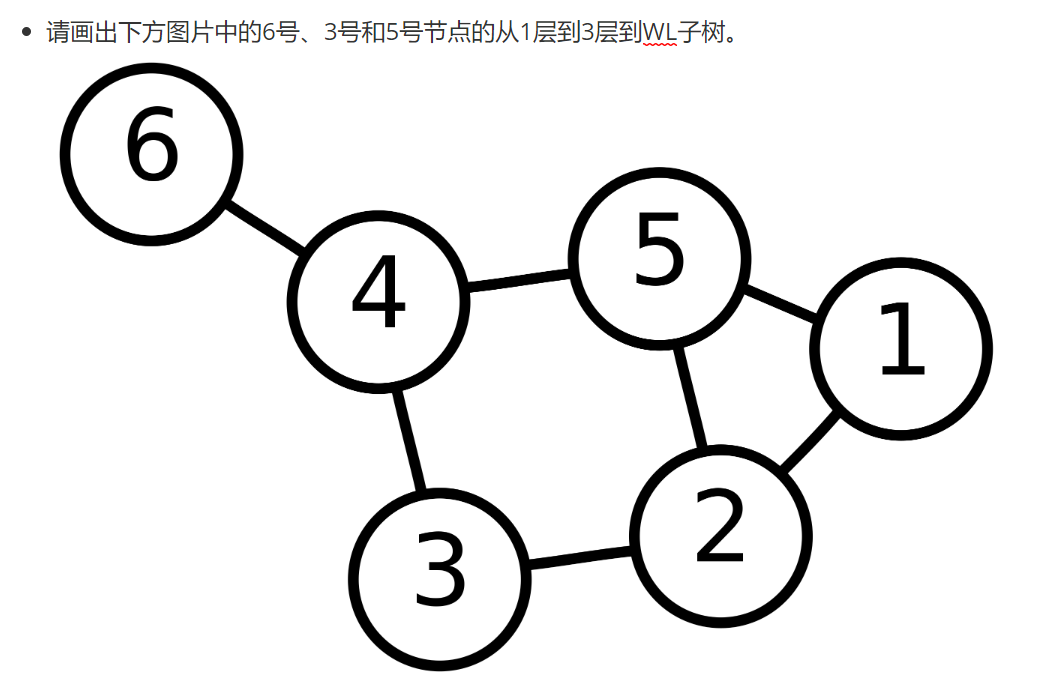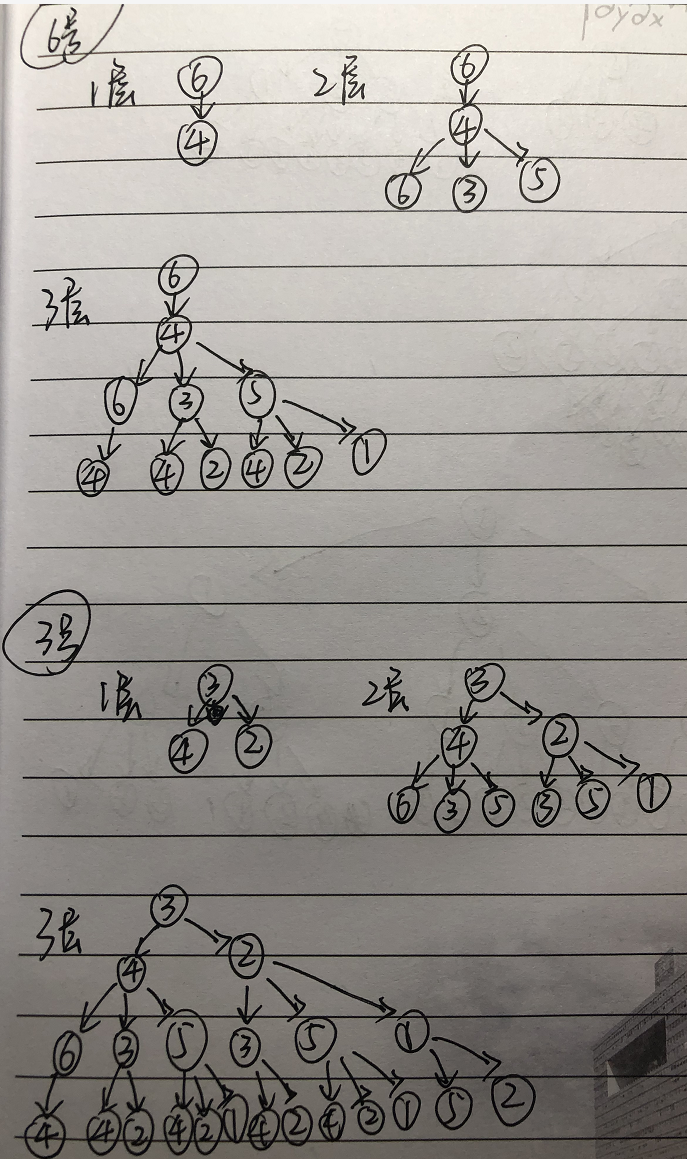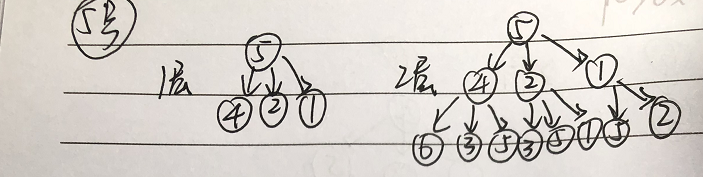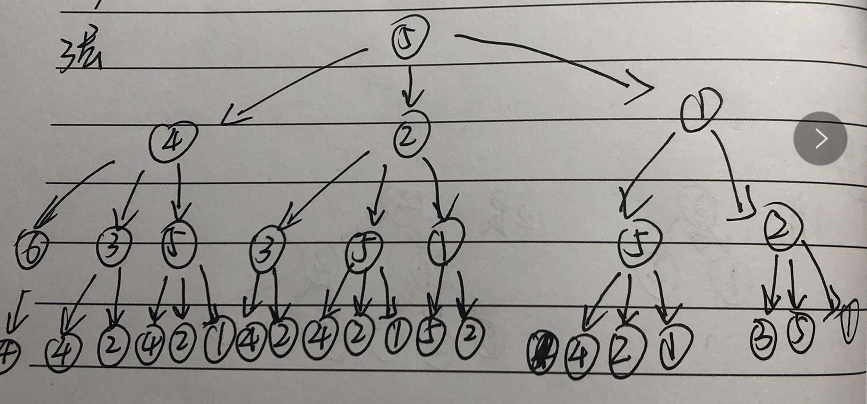# 4 代码实现

## 4.1 基于GIN的图表征模块 GINGraphRepr Module

1. 首先计算得到节点表征

• 代码实现：
• 首先采用GINNodeEmbedding模块对图上每一个节点做节点嵌入（Node Embedding），得到节点表征
• 然后对节点表征做图池化得到图的表征
• 最后用一层线性变换对图表征转换为对图的预测
import torch
from torch import nn
from torch_geometric.nn import global_add_pool, global_mean_pool, global_max_pool, GlobalAttention, Set2Set
from gin_node import GINNodeEmbedding

class GINGraphRepr(nn.Module):

def __init__(self, num_tasks=1, num_layers=5, emb_dim=300, residual=False, drop_ratio=0, JK="last", graph_pooling="sum"):
"""
Args:
num_layers (int, optional): GINConv 层数，默认5
emb_dim (int, optional): 节点维度，默认300
residual (bool, optional): adding residual connection or not. Defaults to False.
drop_ratio (float, optional): dropout rate. 默认0
JK (str, optional): 可选的值为"last"和"sum"。选"last"，只取最后一层的结点的嵌入，选"sum"对各层的结点的嵌入求和。默认"last"
graph_pooling (str, optional): 图池化方式. 可选的值为"sum"，"mean"，"max"，"attention"和"set2set"。 默认 "sum".

Out:
graph representation
"""

super(GINGraphPooling, self).__init__()

self.num_layers = num_layers
self.drop_ratio = drop_ratio
self.JK = JK
self.emb_dim = emb_dim

if self.num_layers < 2:
raise ValueError("Number of GNN layers must be greater than 1.")

self.gnn_node = GINNodeEmbedding(num_layers, emb_dim, JK=JK, drop_ratio=drop_ratio, residual=residual)

# Pooling function to generate whole-graph embeddings
if graph_pooling == "sum":
elif graph_pooling == "mean":
self.pool = global_mean_pool
elif graph_pooling == "max":
self.pool = global_max_pool
elif graph_pooling == "attention":
self.pool = GlobalAttention(gate_nn=nn.Sequential(
nn.Linear(emb_dim, emb_dim), nn.BatchNorm1d(emb_dim), nn.ReLU(), nn.Linear(emb_dim, 1)))
elif graph_pooling == "set2set":
self.pool = Set2Set(emb_dim, processing_steps=2)
else:
raise ValueError("Invalid graph pooling type.")

if graph_pooling == "set2set":
else:

def forward(self, batched_data):
h_node = self.gnn_node(batched_data)

h_graph = self.pool(h_node, batched_data.batch)
output = self.graph_pred_linear(h_graph)

if self.training:
return output
else:
# At inference time, relu is applied to output to ensure positivity
# 因为预测目标的取值范围就在 (0, 50] 内